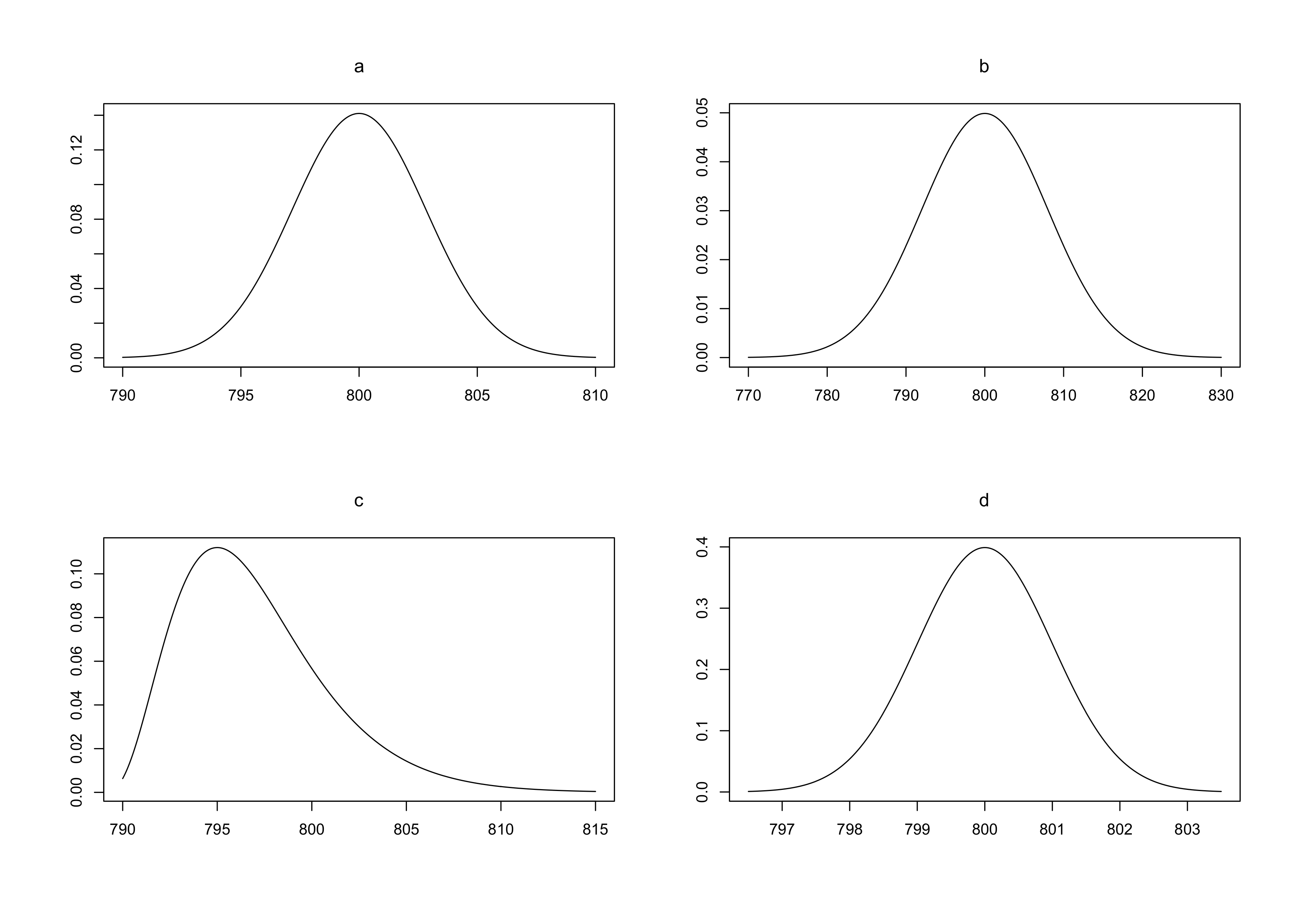0
Correct
0
Incorrect
4
Total

## Question 1 of 4

The change in depth of a river from one day to the next, measured in cm at a specific location, is a continuous uniform random variable $X$ with the following density function: $f(x)=k$ for $-2 \le x \le 2$ and $f(x)=0$ elsewhere.

What is the value of k?

## Question 2 of 4

The change in depth of a river from one day to the next, measured in cm at a specific location, is a continuous uniform random variable $X$ with the following density function: $f(x)=k$ for $-2 \le x \le 2$ and $f(x)=0$ elsewhere.

What is $P(X > 1)$:

## Question 3 of 4

In a consumer survey performed by a newspaper, 20 different groceries (products) were purchased in a grocery store. Discrepancies between the price appearing on the sales slip and the shelf price were found in 6 of these purchased products.

At the same time a customer buys 3 random (different) products within the group consisting of the 20 goods in the store. The probability that no discrepancies occurs for this customer is:

## Question 4 of 4

A package of chocolate consists of 8 chocolate bars. It is assumed that the weight of the individual bars follows a normal distribution with mean weight of $\mu=100$ g and standard deviation $\sigma=1$ g.

Below are figures of four distributions: (a, b, c, and d, from top left to bottom right)Which of the four figures is showing the distribution of the weight of the chocolate packages?